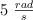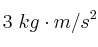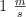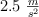# Ejercicios FyQ

Ejercicios Resueltos de Physics & Chemistry (4º ESO)

## Ejercicios de Física y Química para alumnos de 4º de ESO del Programa Bilingüe.

A person of mass 70 kg stands on a weighing scale in an elevator which is moving and observes different lectures on it. Determine the acceleration of the elevator and if it is moving up, down, accelereting, breaking or not moving if the values of the scale are: a) 66 kg, b) 74 kg and c) 70 kg.

The angular velocity of a wheel of a bike is, and its angular acceleration is.

a) What is the angular position and angular velocity at the time t = 5 s?

b) What is the angular position and angular velocity at the time t = 5 s, expressed in revolutions?

c) What is the final velocity and displacement of the bike at t = 5 s if the tire has a diamater of 1 meter?

How many newtons of force are represented by?

A car that starts fromand uniformly accelerates atfor 5 s.

a) Calculate the final velocity and the displacement of the car.

b) What is the diameter of the tire if the angular displacement is 60 rad?

c) What is the final angular velocity and angular acceleration of the tires?

Índice de Ejercicios RESUELTOS

Buscar en esta web

Por palabras clave

Por temas

Búsqueda libre